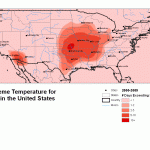# Midrange: Definition and How to Find the Midrange

Statistics Definitions > Midrange

## What is the Midrange?

The midrange is a type of average, or mean. For example, “midrange” electronic gadgets are in the middle-price bracket: not cheap, but not expensive, either.

The formula to find the midrange = (high + low) / 2.

Example problem: Current cell phone prices in a mobile phone store range from \$40 (the cheapest) to \$550 (the most expensive). Find the midrange.

• Step 1: Add the lowest value to the highest: \$550 + \$40 = \$590.
• Step 2: Divide Step 1 by two: \$590 / 2 = \$295.

The mid priced phones would be priced at around \$295.

## Difference Between a Midrange and a Range.

The range is a measure of spread. In the cell phone example, the range would be: \$550 – \$40 = \$510. The range can also mean the entire spread of numbers—for example, it could be written as \$40 to \$550. The mid-range takes it a step further and divides the range by two to find a type of average.

## Difference Between a Midrange and the Interquartile Range.

Don’t confuse the midrange with the interquartile range (IQR), sometimes called the “middle fifty“. They actually mean very different things. The mid-range is a type of mean, while the interquartile range is talking about a chunk of data in the middle of a data set.For example, when the weather service reports that a “mean daily temperature” is 77 degrees, they are talking about the mid-range. They got that number by taking the sum of the high daily temperature and the low daily temperature and dividing by 2. Let’s say the recorded daily temperatures were:
55, 65, 67, 69, 70, 80, 81, 87, 90
High = 90
Low = 55
Mid = (90 + 55) / 2 = 154 / 2 = 77.

The IQR for this data set is the 25th percentile subtracted from the 75th percentile:
25th Percentile: 66
75th Percentile: 84
Interquartile Range: 84 – 66 = 18

Check out our YouTube channel for more stats help and tips!

CITE THIS AS:
Stephanie Glen. "Midrange: Definition and How to Find the Midrange" From StatisticsHowTo.com: Elementary Statistics for the rest of us! https://www.statisticshowto.com/midrange-definition-use/
---------------------------------------------------------------------------Need help with a homework or test question? With Chegg Study, you can get step-by-step solutions to your questions from an expert in the field. Your first 30 minutes with a Chegg tutor is free!